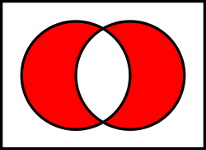Another interesting optimization that I encountered several years ago while a computer science undergraduate at the University of Texas:

How do you efficiently compute the average of two unsigned integers while guaranteeing there is no overflow?

This was actually a question I was asked while interviewing for jobs my senior year. This wasn't the question they started with, and in fact all the previous questions were kind of leading up to this one. The rest seemed a little superfluous, but this one actually has some merit and use, so I wanted to discuss it.

The solution, assuming a and b are the two unsigned integers: ((a ^ b) >> 1) + (a & b)

If bit manipulation is not something you've done before, this might not seem obvious. But if you think about the individual operations, and what they yield, you can start to see how elegantly this works. The right shift by one is clearly a division by two, but how do the other operations fit in? The XOR of a and b can be thought of as a very rough form of addition, one that only works if none of the bits overlap. For example 4 and 8 would XOR nicely into 12, the bit shift would result in a value of 6. 4 & 8 is zero, so the XOR and the shift were all that was needed to give us the average. So clearly the addition at the end of a & b is only to handle the case where XOR fails to properly add the two numbers, which is in fact exactly its purpose.

In the previous example a & b was 0, but consider 4 and 6. 4 ^ 6 >> 1 is 1, which is clearly not the average of 4 and 6. Only after 4 & 6 is added do we get the correct result. Since the overlapping set bits were cleared by XOR, we need a way to properly handle them, which is where the AND operator comes in. If you think about it, its really giving us the average too. In the 4 and 6 example, both numbers have the 3rd bit set, which is 'valued' at 4. XOR omitted each value's 3rd bit from its calculation, essentially depriving the final result of 8 (4 from 4, and 4 from 6). When we AND these two values, we get one of those "4s" back (which is correct since 4 is the average 4 and 4).

Another way to visualize this is with a venn diagram. If you look at what the XOR and AND diagrams show, it makes it even easier to see why the formula is the way it is.You might be wondering why all this is necessary? If you want to avoid a divide by two, why not just bit shift? Well that will not handle the potential overflow from the addition operation! As you've just seen, the operation above is really breaking the number down into two parts and averaging them separately. XOR and bitshift gives us the average of the non-overlapping parts of the number, and the AND operation gives us the average of the overlapping parts of the number. We then combine these and have the complete average without fear of causing an overflow!